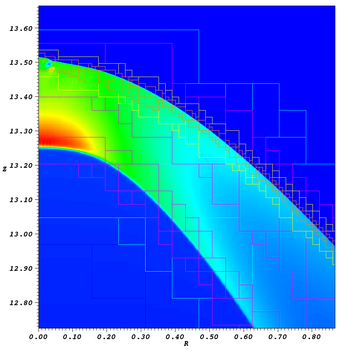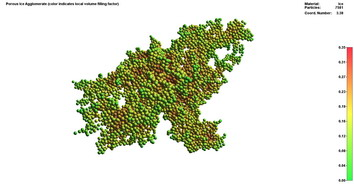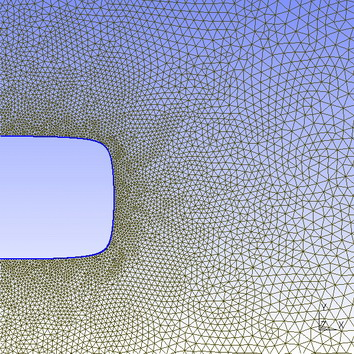# Smoothed Particles Hydrodynamics (SPH)

Ralf Geretshauser, Farzana Meru, Roland Speith (PIT)

SPH is a mesh-free Lagrangian particle method code and was originally developed to simulate astrophysical hydrodynamical scenarios. SPH is closely related to N-body codes in that both are particle codes. While both consider long-range gravity forces, SPH also considers pressure and viscosity. Particle based codes compute variables at a particular spatial point. However, each particle in an SPH code represents a volume of fluid with variables that are representative of the surrounding region (as opposed to the values at a particular point), such as density and pressure. SPH calculates the properties of the volume of fluid (or volume of mass when used for solid body mechanics, see below) by considering the smoothing length of each particle, which essentially calculates an average of a particular quantity. Such properties include the mass, momentum, energy and velocity, to name a few.

SPH is able to provide accurate results for problems involving strong shocks and complex geometries. In addition, the computational time is significantly reduced in low density regions allowing more computational time to be focussed on high density regions. Furthermore, SPH deals with free boundaries much more easily than grid-based codes.

Originally designed for the simulation of compressible flows in astrophysical contexts, SPH was enhanced in the past decades and is nowadays applicable for a variety of physical problems. By including stress-strain relations this scheme can be expanded for the simulation of solid bodies instead of flows and fluids. First, solid body SPH was applied in hyper-velocity collisions and explosions for military and industrial purposes. Later its suitability for impact simulations became valuable also in an astrophysical context: a model for brittle fracture has been implemented, which made it possible to simulate collisions between rocky asteroids. This model was applied to study the formation of asteroid families in high-velocity impacts. Under the assumption that planetesimals consist of rock-like material, planetesimal collisions were also simulated. All these simulations did not involve the treatment of porosity. Later, on a model with porosity-dependent strength quantities was proposed which was applied to the collision of ice aggregates. Based on a different porosity model, SPH has been expanded for the simulation of porous rock-like brittle material. Again, asteroid collisions and the influence of porosity were studied.

In our group we use a three-dimensional SPH code to study collisions of dust aggregates to understand under what conditions dust aggregates may grow to become planetesimals. In addition, we use a three-dimensional SPH code to simulate the fragmentation of self-gravitating discs.

Bertram Bitsch, Philipp Buchegger, Markus Flaig, Willy Kley, Stefan Kolb, Farzana Meru, Tobias Müller, Matthias StuteSimulation of the bow shock of a propagating jet; also shown are the grid levels of adaptive mesh refinement (AMR)

Grid-based computational simulations rely on a grid underlying the physical process. The simulated accretion disc is therefore divided into different grid cells, each having a specific length, width and height. These cells form the grid, in which the hydrodynamic equations are solved.

Hydrodynamics deals with the fluid flow of liquids and gases. In our case, we are interested in the motion of gas e.g. in an accretion disc around young stellar objects. To determine the flow, the Navier-Stokes equations are solved. A reasonably easy way to do this is to parameterise the accretion disc into grid cells, where each grid cell has a given temperature, gas density, pressure and so on. The flow from each grid cell to the neighbouring grid cells can be determined and thus a general flow pattern of gas in the accretion disc can be obtained.

Magnetic fields are supposed to play an important role in the dynamics of accretion discs. In order to include the effects of magnetic fields, we have to add the Lorentz force and also the induction equation (i.e. Amperes law), which determines the evolution of the magnetic field. This leads to the equations of magneto-hydrodynamics (MHD), which in most cases can only be solved numerically. In numerical simulations, special care has to be taken in order to prevent the occurence of magnetic monopoles, which can render the results unphysical or even cause the code to crash. Since protoplanetary discs are for a large part only poorly ionised, we also have to include non-ideal MHD effects, like Ohmic diffusivity.

As gas inside a disc produces viscous heating and photons transport the heat from the midplane of the disc to the upper layers of the disc, radiation of these photons has to be taken into account. For this purpose, the inclusion of radiation using the flux-limited-diffusion approximation (an approximation that allows us to deal with the transition between optically thick and thin media) into the equations is neccessary. This diffusion equation also has to be taken into account in the Navier-Stokes equations.

# Molecular Dynamics

Simeon Carstens, Willy Kley, Alexander SeizingerSimulation of porous ice agglomerates

To study the behaviour of particle agglomerates we use a molecular dynamics approach, which means that the motion of each monomere is calculated individually. In our model we use short range particle interaction. Thus, to determine the force or torque acting upon a specific particle only particles that are in contact with it need to considered.

A typical feature of a molecular dynamic codes is the huge amount of fine grained parallelism, as for example all particle positions can be updated in parallel after the current velocity and acceleration have been determined. To take adantage of this we want to parallelize our code using GPU computing techniques such as CUDA.

# Finite Element Method (FEM)